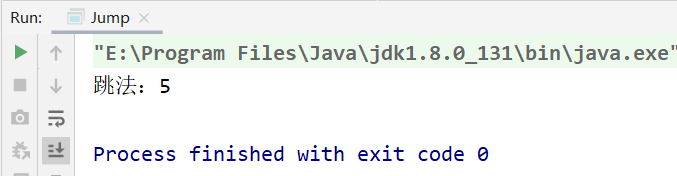• 求该青蛙跳上一个n级的台阶总共有多少种跳法（先后次序不同算不同的结果）。 思路： 斐波那契数列变体，关键是找出递推公式。 假设跳n级台阶有f(n)中跳法，容易发现f(1)=1,f(2)=2; n&gt;2时，如果最后一次跳...
I 题目：

一只青蛙一次可以跳上1级台阶，也可以跳上2级。求该青蛙跳上一个n级的台阶总共有多少种跳法（先后次序不同算不同的结果）。

思路：

斐波那契数列变体，关键是找出递推公式。

假设跳n级台阶有f(n)中跳法，容易发现f(1)=1,f(2)=2; n>2时，如果最后一次跳一级台阶，一共有f(n-1)种跳法，如果最后一次跳两级台阶，一共有f(n-2)种跳法。即f(n)=f(n-1)+f(n-2)。

代码：

在线测试OJ：

https://www.nowcoder.com/practice/8c82a5b80378478f9484d87d1c5f12a4?tpId=13&tqId=11161&rp=1&ru=/ta/coding-interviews&qru=/ta/coding-interviews/question-ranking

AC代码：

class Solution {
public:
//循环
int jumpFloor(int number) {
if(number <= 0)
return 0;
if(number == 1)
return 1;
if(number == 2)
return 2;
int n_1 = 2;
int n_2 = 1;
int f_n;
for(int i = 3; i <= number; i++)
{
f_n = n_1 + n_2;
n_2 = n_1;
n_1 = f_n;
}
return f_n;
}
};

II 题目：

一只青蛙一次可以跳上1级台阶，也可以跳上2级……它也可以跳上n级。求该青蛙跳上一个n级的台阶总共有多少种跳法。

思路：

斐波那契数列变体，关键是找出递推公式。

同样的思路，但是递推公式变为，f(1)=1,f(2)=2,f(n)=1+f(1)+f(2)+...+f(n-2)+f(n-1)，由f(n-1)=1+f(1)+f(2)+...+f(n-2)，两式相减可得，f(n)=2f(n-1),n>=3。用数学归纳法可以证明，f(n)=2^(n-1)。

代码：

在线测试OJ：

https://www.nowcoder.com/practice/22243d016f6b47f2a6928b4313c85387?tpId=13&tqId=11162&rp=1&ru=/ta/coding-interviews&qru=/ta/coding-interviews/question-ranking

AC代码：

class Solution {
public:
int jumpFloorII(int number) {
if(number <= 0)
return 0;
if(number == 1)
return 1;
if(number == 2)
return 2;
int n_1 = 2;
int f_n;
for(int i = 3; i <= number; i++)
{
f_n = 2*n_1;
n_1=f_n;
}
return f_n;
}
};


展开全文• 首先青蛙跳台阶大致分为三个难度模式，这个里边所谓的公式不要去背，建议将多项式列出来，自己化简就行了。下边一一介绍这三个版本： 1.初级版： 告诉给定的台阶数，并且题目交代：青蛙只能一次跳一个台阶或者青蛙跳...
一、青蛙跳台阶不同难度的介绍
首先青蛙跳台阶大致分为三个难度模式，这个里边所谓的公式不要去背，建议将多项式列出来，自己化简就行了。下边一一介绍这三个版本：
1.初级版：
告诉给定的台阶数，并且题目交代：青蛙只能一次跳一个台阶或者青蛙跳两个台阶。这个题我们在简单的列举之后会发现台阶的高度和青蛙的跳法是有规律的（和斐波那契数列很相似，只是换掉了第二项，构成次序列的思路与斐波那契数列完全一样）。
下边是具体的代码（内部含有详细的思路和化简说明）
// 1.简单的跳台阶：说给定一个n阶的台阶，青蛙一次只能挑一个或者两个，问有多少种跳法
// 结论：最后转换和斐波那契数列相似，局部变化了
// 列举：一个台阶:(1)  一种
//      两个台阶:(1,1),(2) 两种
//      三个台阶:(1,2),(2,1),(1,1,1) 三种
//      四个台阶:(2,2),(1,2,1),(1,1,2),(2,1,1),(1,1,1,1) 四种 ......
// 通过上边的列举我们可以看见，这就是跟斐波那契的思路一样了，只不过第二项发生了变化
// 后边值 = 前一项 + 前前一项，下边是初阶跳台阶的写法哈

public static int primaryJump(int n) {// 方法里边传的参数是台阶的数
// 斐波那契得用递归，简单
// 特殊情况处理
if (n == 0) {
return 0;
}
if (n == 1) {
return 1;
}
// 这里的第二项要处理一下，因为这里的2不是0项和1项的和，而斐波那契中就不用处理，
// 它满足那个关系
if (n == 2) {
return 2;
}
// 一般情况处理，递归处理
return primaryJump(n - 1) + primaryJump(n - 2);
}

2.中级版：
告诉台阶数了，这里变化的是青蛙能跳的台阶的数不在是1或者2，跳法而是从1~n阶，从一次一个台阶到一次直接蹦完所有的台阶，直接登顶。这个题我们可以借鉴初级版的一些结论。
下边是具体代码的实现过程（内部含有详细解释和推导）
// 2.困难的青蛙跳台阶，说是给定n个台阶，这个青蛙可以一次可以跳1,2,3,4,5... n个台阶。
// 刚已经分析了，一次跳一阶，返回的是primaryJump(n - 1)，两次是primaryJump(n - 2)
// 那么结论： n阶就是primaryJump(n - n)。然后把所有的每一次都递归的加起来即可
// 经过列举观察可发现： primaryJump(n) = 2*primaryJump(n - 1)
// 那么这里跳的台阶的跳法就是：(n - n) + (n - (n - 1)) + (n- (n - 2))....(n - 1)
//              对应的就是：  (0) + (1) + (2) + .....(n - 1)

public static int difficultJump(int n) {
// 特殊情况的处理
if (n == 0) {
return 0;
}
if (n == 1) {
return 1;
}
// 一般情况的处理，带那个公式进行递归即可
return 2 * difficultJump(n - 1);
}

3.变态版：
在中级版的基础上进行了改造，给定的要上的台阶的最大高度和青蛙每一次能上的台阶数是未知的。我们不知道到底是台阶最大高度大还是青蛙每次能跳的最大高度大。这里就要比较一下，具体情境具体处理即可。
下边是代码实现过程（内含有详细的注解和推导）
// 3.变态跳台阶：给定的台阶数和青蛙跳的最大的台阶数不一样。
// 题目要求：说一个青蛙能跳的台阶是1,2,3,4,....n,现在给定一个m级的台阶要青蛙跳，
// 有多少种跳法
// 分为两种情况：(1)要是给的台阶的高度m小于青蛙能跳的最大台阶数n，青蛙能从1阶跳到m阶
//             (2)要是给定的台阶高度m大于等于能跳的最大台阶数n,青蛙能从1阶跳到n阶
// f(n) = f(n-1) + f(n-2) +..... + f(n-m);
// f(n-1) = f(n-2) + f(n-3) + .....+ f(n-m-1);
// 这里化简两个式子可得到一个关系式 f(n) = 2*f(n-1) - f(n-m-1);
// 下边是具体的代码操作。
public static int pervertedJump(int n,int m) {
// n表示的是青蛙能跳的最大台阶数，m表示的是给定的台阶的高度

// (1)台阶高度<最大能跳台阶数，能跳的是(1~m),注意里边要填的参数
if (m < n) {
return 2*pervertedJump(n - 1,m) + pervertedJump(n - m - 1,m);
}

// (2)台阶高度>=最大能跳台阶数,跳的是(1~n)，注意里边要填的参数。
if (n <= 1) {
return 1;
} else {
return 2*pervertedJump(n - 1,n);
}
}



展开全文• 求该青蛙跳上一个n级的台阶总共有多少种跳法。 例如：0 1 2 3 4；n=4 跳法：1111，121，211，112，22 求和：5种 递推公式：f(n)=f(n-1)+f(n-2) public class Jump { public static void main(String[] args) { int...
问题描述： 一只青蛙一次可以跳上1级台阶，也可以跳上2级。求该青蛙跳上一个n级的台阶总共有多少种跳法。
例如：0 1 2 3 4；n=4
跳法：1111，121，211，112，22
求和：5种
递推公式：f(n)=f(n-1)+f(n-2)
public class Jump {
public static void main(String[] args) {
int target=4;
int sum = fun(target);
System.out.println("跳法总数："+sum);
}
public static int fun(int target){
if(target<=0) return 0;
if(target==1) return 1;
if(target==2) return 2;
return fun(target-1)+fun(target-2);
}
}

运行结果展开全文算法
• 变态青蛙跳台阶青蛙跳台阶问题的一种变形，不过是加上了从任意一层台阶跳上去，这样我们的状态转移公式就变成了 f(n) = f(n-1) + f(n - 2) + f(n-3)+ ***** + f(1) 根据数学推导很容易得出f(n-1) = f(n-2) + f(n...
题目描述

一只青蛙一次可以跳上1级台阶，也可以跳上2级……它也可以跳上n级。求该青蛙跳上一个n级的台阶总共有多少种跳法。

变态青蛙跳台阶是青蛙跳台阶问题的一种变形，不过是加上了从任意一层台阶跳上去，这样我们的状态转移公式就变成了

f(n) = f(n-1) + f(n - 2) + f(n-3)+ ***** + f(1)

根据数学推导很容易得出f(n-1) = f(n-2) + f(n-3) + ***** + f(1)

所以f(n) = f( n - 1)

class Solution {
public:
int jumpFloorII(int number) {
long long v[number];
v = 0;
v = 1;
for(int i = 2;i <= number; i++)
{
v[i] = 2*v[i - 1];
}
return v[number];
}
};


展开全文• 笔试算法：青蛙跳台阶 1、题目表述 //假设青蛙正在跳台阶。需要 n 阶你能到达楼顶。 //每次青蛙可以跳 1 或 2 个台阶，但不可以连续跳2个。请问有多少种不同的方法可以到楼顶呢？ //注意：给定 n 是一个正整数。 2、...算法 动态规划
• 青蛙跳台阶问题1：一次只能跳1个或2个台阶。青蛙跳到台阶为n的地方有多少种方式。 一次跳1或2,f(n)表示n个台阶一次跳1或2的可能情况 那么f(n)= 最后一次跳1层到n了 + 最后一次跳2层到n了 的情况和 所以 f(n) = f(n-...
• 08 图解剑指Offer 青蛙跳台阶 Java题解08 图解剑指Offer 青蛙跳台阶 Java题解题目链接题目描述题解:图解:代码:复杂度 08 图解剑指Offer 青蛙跳台阶 Java题解 题目链接 题目描述 一只青蛙一次可以跳上1级台阶，也可以...算法 数据结构 leetcode 动态规划 斐波那契数列
• 求该青蛙跳上一个n 级的台阶总共有多少种跳法。 我的思路：最开始我的思路是把这个看成是一个数学问题，n=i*1+k*2先把所有可能满足这个公式的i和k求出来。然后在对i和k做排列组合。很明显i的范围应该是0,所以我们已i...
• 求该青蛙跳上一个n级的台阶总共有多少种跳法（先后次序不同算不同的结果）。 分析： 穷举找规律法： 1------1 2------2 3------3 4------5 题解： 方法一： 由此找通项公式，即：F(n) = F(n-1)+F(n-2) 大家可以看出...算法
• 文章目录青蛙跳台阶汉诺塔具体流程图提示总结 青蛙跳台阶 这个篇幅不多比较简单汉诺塔问题比较简单 青蛙跳台阶思想 青蛙跳台阶和斐波那契数列基本一样 区别多了几个判断 看这个图就可以推导出来公式Fib（n-1）+Fib...
• 2.青蛙最后一步要么跨1个台阶要么跨2个台阶。 3.当最后一步跨1个台阶时即之前有n-1个台阶，根据1的假设即n-1个台阶有f(n-1)种走法。 4. 当最后一步跨2个台阶时即之前有n-2个台阶，根据1的假设即n-2个台阶有f(n-2 )种...
• 《剑指offer》编程-斐波那契数列/青蛙跳台阶 #情景：一只青蛙一次可以跳上1级台阶，也可以跳上2级。求该青蛙跳上一个n级的台阶总共有多少种跳法（先后次序不同算不同的结果）/ 斐波那契数列问题 斐波那契数列递推...斐波那契数列
• 看到题目第一个想法就是递归，假设青蛙跳上n级台阶有f(n)种可能的方法，可以分成两大类情况。第一种是最后一次跳了一级台阶，共有f(n-1)种，第二种是最后一次跳了两级台阶，这种方法共有f(n-2)种。得出递推公式f(n)=...
• 斐波拉契数列，f(n)= f(n-1)+f(n-2)递推 ，上一个台阶有一种方法，上个台阶有两种。之后用公式递推即可，属于找规律型题目。 其实也可以从后面开始考虑，1个台阶跳到终点有1 种，2个有两种跳法。递推公式取决于...
• 青蛙跳n个台阶，问有几种跳法。 题上说青蛙一次可以跳一级或者两级台阶。那么跳n个台阶的跳法是不是就等于 跳到n-1级的跳法和跳到n-2级的跳法之和呢。 也就是numWays(n) =numWays(n-1)+numWays(n-2); 有这个递推...
• 1.青蛙跳台阶问题：一只青蛙一次能跳1个台阶或2个台阶，问：跳上第n阶台阶共有几种方法？ 首先可以知道跳1阶只要1种方法，跳2阶有2种方法；跳到第n阶，必定是从n-1阶跳上去，或者从n-2阶跳上去，定义一个函数jump，...
• 求该青蛙跳上一个 n 级的台阶总共有多少种跳法。 答案需要取模 1e9+7（1000000007），如计算初始结果为：1000000008，请返回 1。 来源：力扣（LeetCode） 方法1 递归 思路： 递推公式为f(n)=f(n-1)+f(n-2)。利用...leetcode 动态规划
• 青蛙跳上 n 级台阶有 f(n)种方法，把这 n 种方法分为两大类，第一种最后一次跳了一级台阶，这 类方法共有 f(n-1)种，第二种最后一次跳了两级台阶，这种方法共有 f(n-2)种，则得出递推公式 f(n)=f(n-1)+f(n-2)，...
• 有助于目录的生成如何改变文本的样式插入链接与图片如何插入一段漂亮的代码片生成一个适合你的列表创建一个表格设定内容居中、居左、居右...列表如何创建一个注脚注释也是必不可少的KaTeX数学公式新的甘特图功能...Java
• 求该青蛙跳上一个 n 级的台阶总共有多少种跳法。 答案需要取模 1e9+7（1000000007），如计算初始结果为：1000000008，请返回 1。 动态规划 动态规划算法通常基于一个递推公式及一个或多个初始状态。当前子问题的解将...
• 求该青蛙跳上一个n级的台阶总共有多少种跳法。 其实题目很水...就是一个等比数列通项公式嘛 f(0)=1 f(1)=1 f(n)=f(0)+f(1)+...+f(n-1) ==> f(n)=2*f(n-1) (when n>=2) ==> f(n)=2^(n-1) cla...
• 题目解析3.1 方法一：自下而上、循环求解3.2 青蛙跳台阶、经典递归3.3 fib数列O(logn)O(logn)O(logn)计算法3.4 斐波拉契数列通项公式与求和公式 1. 题目来源 链接：用两个栈实现队列 来源：LeetCode——《剑指-...递归
• 1. 题目描述 大家都知道斐波那契数列，现在要求输入一个整数n，请你输出斐波那契数列的第n项（从0开始，第0项为0...斐波那契数列公式: f(n)=0 n=0 f(n)=1 n=1 f(n)=f(n-1)+f(n-2) n>1 class Solution { ......